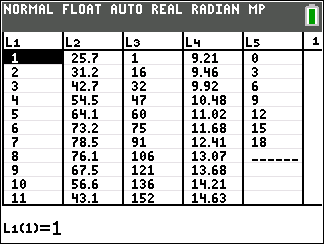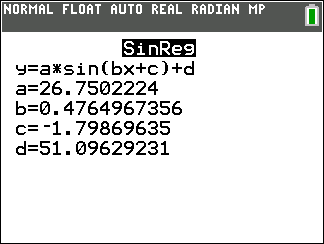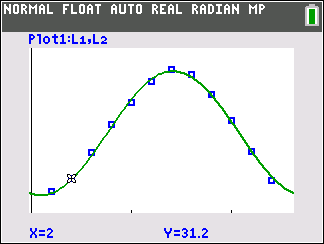### Mathematics lessons for IB® Diploma Programme

Applications and Interpretation | Geometry and Trigonometry## Find That Sine

### Activity Overview

Sinusoidal regression is used to determine equations to model various data sets, and the equations are used to make inferences.

## Key Steps## Step 1

Students explore sinusoidal regression by determining and graphing the sine regression equation.

Students will use the program KANSTEMP which will load six lists with data for all three problems.## Step 2

They use data for the average monthly temperature for Kansas City to determine the sine equation that models the data.

Students produce a scatter plot with the data given to them in their spreadsheet and find the sine equation that models the data.## Step 3

In Problem 2, students will then find the minimum and maximum points on the curve for four dates. These points will represent the summer and winter solstices.